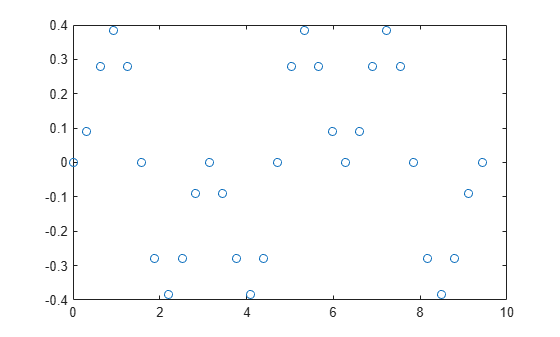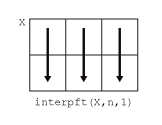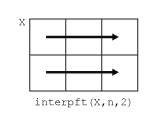# interpft

## 语法

``y = interpft(X,n)``
``y = interpft(X,n,dim)``

## 说明

``y = interpft(X,n)` 在 `X` 中内插函数值的傅里叶变换以生成 `n` 个等间距的点。`interpft` 对第一个大小不等于 1 的维度进行运算。`

``y = interpft(X,n,dim)` 沿维度 `dim` 运算。例如，如果 `X` 是矩阵，`interpft(X,n,2)` 将在 `X` 行上进行运算。`

## 示例

```dx = 3*pi/30; x = 0:dx:3*pi; f = sin(x).^2 .* cos(x); plot(x,f,'o')``````N = 200; y = interpft(f,N);```

```dy = dx*length(x)/N; x2 = 0:dy:3*pi; y = y(1:length(x2));```

```hold on plot(x2,y,'.') title('FFT Interpolation of Periodic Function')``````A = randn(3,20); x = 1:20;```

```N = 500; y = interpft(A,N,2);```

```dy = length(x)/N; x2 = 1:dy:20; y = y(:,1:length(x2));```

```subplot(3,1,1) plot(x,A(1,:)','o'); hold on plot(x2,y(1,:)','--') title('Row 1') subplot(3,1,2) plot(x,A(2,:)','o'); hold on plot(x2,y(2,:)','--') title('Row 2') subplot(3,1,3) plot(x,A(3,:)','o'); hold on plot(x2,y(3,:)','--') title('Row 3')```## 输入参数

• `interpft(X,n,1)``X` 的列进行插值。• `interpft(X,n,2)``X` 的行进行插值。## 算法

`interpft` 函数使用 FFT 方法。使用 `fft` 将原始向量 `x` 变换为傅里叶域，然后通过更多的点变换回原始向量。